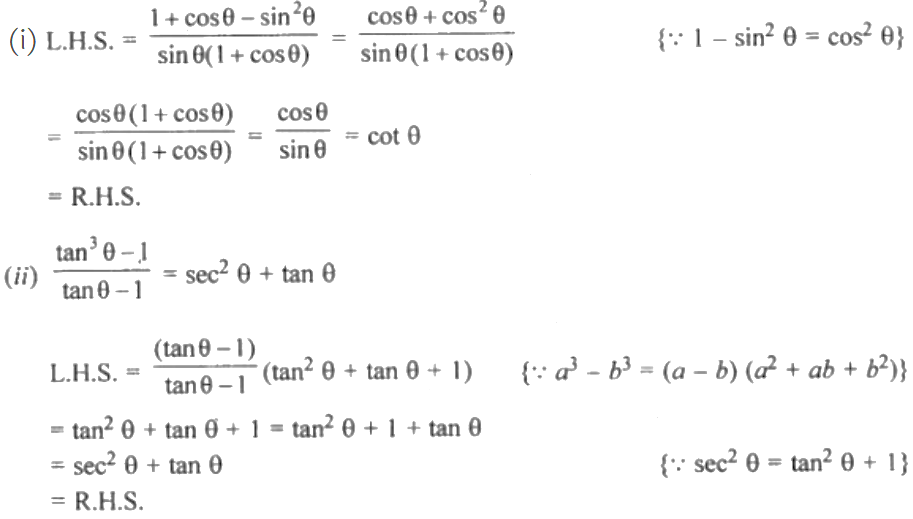Guru

# (i) (1+cos theta -sin^2 theta) / sin theta (1 + cos theta ) = cot theta. (ii)tan³ A – 1 / tan A -1 = sec² A + tan A

• 0

This ques has been taken from the Book Book- ML Aggarwal
Board- ICSE
Publication- Avichal
Chapter- Trigonometric Identities
Chapter number-18
This is an important question and asked in previous exams.

(i) (1+cos theta -sin^2 theta) / sin theta (1 + cos theta ) = cot theta. (ii)tan³ theta- 1 / tan theta -1 = sec² theta+ tan theta

Class 10, chapter 18,  ML aggarwal, trigonometric identities, question no 21

Share